Courses

# Quant Mock Test

## 20 Questions MCQ Test Mock Test Series for CLAT 2020 | Quant Mock Test

Description
This mock test of Quant Mock Test for CLAT helps you for every CLAT entrance exam. This contains 20 Multiple Choice Questions for CLAT Quant Mock Test (mcq) to study with solutions a complete question bank. The solved questions answers in this Quant Mock Test quiz give you a good mix of easy questions and tough questions. CLAT students definitely take this Quant Mock Test exercise for a better result in the exam. You can find other Quant Mock Test extra questions, long questions & short questions for CLAT on EduRev as well by searching above.
QUESTION: 1

### How much above the cost price should a man mark his goods, so that, after allowing a discount of 10% for cash payment, he may still make a profit of 8%?

Solution: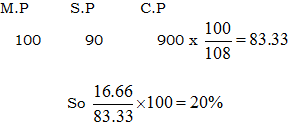QUESTION: 2

### Two numbers are respectively 20% and 50% more than a third number. These two numbers are in the ratio:

Solution: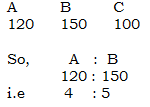QUESTION: 3

### If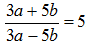, then a : b is equal to:

Solution:

Go via options (d) option satisfies it.

QUESTION: 4

The ratio of the present ages of puneet and Appu is 2 : 3. After 3 years the ratio of their ages will be 3 : 4. The present age of Puneet is:

Solution:

Ratio (1) = 3 yrs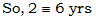QUESTION: 5

Annual incomes of A and B are in the ratio 4 : 3 and their annual expenses are in the ratio 3 : 2. If each of them saves Rs. 600 at the end of the year, what is the annual income of A?

Solution: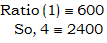QUESTION: 6

The average of marks in Mathematics for 5 students was found to be 50. Later, it was discovered that in the case of one student the marks 48 were misread as 84. The correct average is:

Solution:

Out – 84
In  - 48
Diff = 36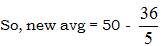= 42.8

QUESTION: 7

If the cost price of 10 articles is equal to the selling price of 7 articles, then the gain or loss per cent is:

Solution:

C.P (10) = S.P (7)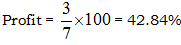QUESTION: 8

Two bicycles were sold for Rs. 3990 each, gaining 5% on one and losing 5% on other. The gain or loss per cent on the whole transaction is:

Solution: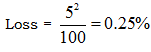QUESTION: 9

Two trains are running with speeds 30 km/hr and 58 km/hr in the same direction. A man in the slower train passes the faster train in 18 seconds. The length (in metres) of the faster train is:

Solution: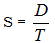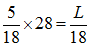So, L = 140 m

QUESTION: 10

In a school 40% of the students play football and 50% play cricket. If 18% of the students neither play football nor cricket, the percentage of the students playing both is:

Solution:

F + C = 90%
But, 100% - 18% = 82%
So, Diff = 90% - 82% = 8%

QUESTION: 11

If the side of a square is increased by 25%, then its area is increased by:

Solution: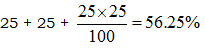QUESTION: 12

A student has to secure 40% marks to pass. He gets 90 marks and fails by 10 marks. Maximum marks are:

Solution: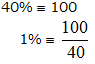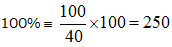QUESTION: 13

If A’s height is 10% more than B’s height, by how much per cent less is B’s height than that of A?

Solution: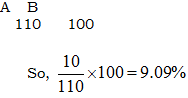QUESTION: 14

A reduction of 20% in the price of sugar enables a purchaser to obtain 3 kg more for Rs. 120. The original price (per kg) of sugar is:

Solution:

Reduction = 20%
Increase in quantity = 1/4
So 1/4 = 3 kg
So, original price = 120/12 = 10

QUESTION: 15

If the difference between the simple and compound interests on a sum of money for 2 years at 4% per annum is Rs. 80, the sum is:

Solution: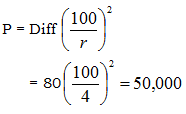QUESTION: 16

A number, when divided by 119, leaves a remainder of 19. If it is divided by 17, it will leave a remainder of:

Solution: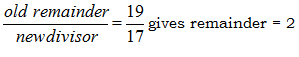QUESTION: 17

The L.C.M. of two numbers is 20 times their H.C.F. The sum of H.C.F. and L.C.M. is 2520. If one of the numbers is 480, the other number is:

Solution:

L x H = a x b
20 H x H = 480 x b
L + H = 2520
So, b = 600

QUESTION: 18

The number, whose square is equal to the difference between the squares of 975 and 585, is:

Solution:

x2 = (975)2 – (585)2
x2 = 608400
So, x = 780

QUESTION: 19

A tap can fill a cistern in 8 hours and another tap can empty it in 16 hours. If both the taps are open, the time (in hours) taken to fill the tank will be:

Solution: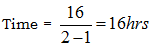QUESTION: 20

Out of 10 teachers of a school, one teachers retires and in his place a new teacher of age 25 years joins. As a result of it, the average age of the teachers is reduced by 3 years. The age of the retired teacher is

Solution:

Age = 25 + 3(10)
= 55 years